### EWMAARL Function

computes the average run length for an exponentially weighted moving average.

#### Syntax

EWMAARL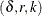where
is the shift to be detected, expressed as a multiple of the process standard deviation (), where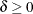. r is the weight factor for the current subgroup mean in the EWMA, where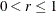. If r = 1, the EWMAARL function returns the average run length for a Shewhart chart for means. Refer to Wadsworth, Stephens, and Godfrey (1986). If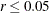,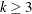, and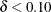, the algorithm used is unstable. However, note that the EWMA behaves like a cusum when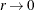, and in this case the CUSUMARL function is applicable. k is the multiple ofused to define the control limits, where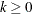. Typically k = 3.

#### Description

The EWMAARL function computes the average run length for an exponentially weighted moving average (EWMA) scheme using the method of Crowder (1987a, 1987b). The notation used in the preceding list is consistent with that used in the MACONTROL procedure.

For a specified shift, you can use the EWMAARL function to design an exponentially weighted moving average scheme by first calculating average run lengths for a range of values of r and k and then choosing the combination of r and k that yields a desired average run length.

#### Examples

The following statements specify a shift of 1, a weight factor of 0.25, and 3control limits. The EWMAARL function returns an average run length of 11.154267016.

data;
arl=ewmaarl(1.00,0.25,3.0);
put arl;
run;